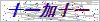# DN20内环型金属环绕垫

## 简要描述：

DN20内环型金属环绕垫 金属带为预成型的V型或W型窄带，表面应光滑.洁净，不应有粗糙不平.裂纹.划伤.凹坑及锈斑等缺陷

DN20内环型金属环绕垫

DN20内环型金属环绕垫

DIM(mm)ANSI B16.5BS1560(mm)Mss sp-44API605公差内环内径垫片内径<=600<=24" >=26" and <=34"±0.5>600 and <=800>24" and <=34>=36" and <=60"±0.9>800 and <=1600 >34" and <=60" ±1.3>1600>60" ±1.6垫片外径<=600<=24"<=24"±0.9>600 and <=160000 >24" and <=60" ±1.81600>60" ±2.2外环外径 >1/2" and <=60"±0.9<=600<=24" 0-1.0 >600 and <=1000>24" and <=34" 0-1.8>1000 and <=1600>34" and <=60" 0-2.2注:另外，我们也可以根据JIS,JPI标准或客户要求生产### 留言框

• #### 验证码：请输入计算结果（填写阿拉伯数字），如：三加四=7

QQ在线客服

15932643382

0316-5951403